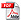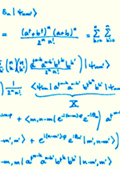Unifying parameter estimation and the Deutsch-Jozsa algorithm for continuous variables

Marcin Zwierz, Carlos A. Pérez-Delgado and Pieter Kok

Physical Review A 82, 042320 (2010)We reveal a close relationship between quantum metrology and the Deutsch-Jozsa algorithm on continuous variable quantum systems. We develop a general procedure, characterized by two parameters, that unifies parameter estimation and the Deutsch-Jozsa algorithm. Depending on which parameter we keep constant, the procedure implements either the parameter estimation protocol or the Deutsch-Jozsa algorithm. The parameter estimation part of the procedure attains the Heisenberg limit and is therefore optimal. Due to the use of approximate normalizable continuous variable eigenstates the Deutsch-Jozsa algorithm is probabilistic. The procedure estimates a value of an unknown parameter and solves the Deutsch-Jozsa problem without the use of any entanglement.##### Teaching

© 2019 Pieter Kok